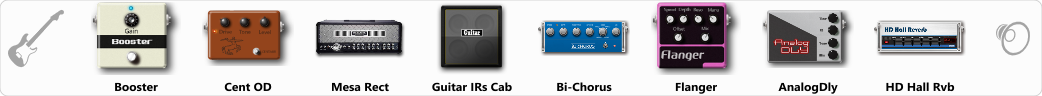# Dream 80´s Guitar FX

Discussion in 'ToneLib-GFX presets' started by Lester Falcon, Aug 11, 2019.

1. Dream 80´s Guitar FX

Preset name: Dream 80´s Guitar FX

IM very bad making tones but this is a good way to practice, soo leave me acomment.

Effects chain:Effect: "Booster" (Dynamics / Filter), active - "yes"
"Gain" = 35

Effect: "Cent OD" (Overdrive / Distortion), active - "yes"
"Drive" = 8
"Tone" = 49
"Level" = 50

Effect: "Mesa Rect" (Amp simulators), active - "yes"
"Gain" = 50
"Bass" = 30
"Middle" = 65
"Treble" = 50
"Presence" = 50
"Master" = 50
"Output" = 50
"Level (dB)" = 5

Effect: "Guitar IRs Cab" (Cabinets), active - "yes"
"Model" = Fender Deluxe Rev (1x12")
"Mic Position" = Middle
"Mic Distance" = Near
"Low Cut (Hz)" = 60
"Hi Cut (kHz)" = 20.0
"Mix" = 100
"Level (dB)" = 0

Effect: "Bi-Chorus" (Modulation / Sfx), active - "yes"
"Speed A" = 3.2
"Speed B" = 4.0
"Depth" = 35
"Reso" = 10
"Mode" = Parallel
"Mix" = 100

Effect: "Flanger" (Modulation / Sfx), active - "yes"
"Speed" = 0.6
"Depth" = 71
"Reso" = 21
"Center" = 7.7
"Offset" = 0.0
"Mix" = 40
"Reset" = On

Effect: "AnalogDly" (Delay), active - "yes"
"Time" = 380
"Feedback" = 34
"Tone" = 65
"Mix" = 65

Effect: "HD Hall Rvb" (Reverb), active - "yes"
"Time" = 7.7
"PreLPF" = 44
"PreDelay" = 96
"HiDamp" = 27
"LoGain" = -3.3
"Mix" = 48

Note: You will need to download and install the ToneLib-GFX software to use the preset.

File size:
692 bytes
Views:
10,535